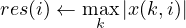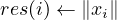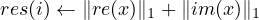#### 4.7 psb_geasums — Generalized 1-Norm of Vector

This subroutine computes a series of 1-norms on the columns of a dense matrix x:This function computes the 1-norm of a vector x.
If x is a real vector it computes 1-norm as:else if x is a complex vector then it computes 1-norm as:call psb_geasums(res, x, desc_a, info)

 res x Subroutine Short Precision Real Short Precision Real psb_geasums Long Precision Real Long Precision Real psb_geasums Short Precision Real Short Precision Complex psb_geasums Long Precision Real Long Precision Complex psb_geasums

Table 7: Data types

Type:
Synchronous.
On Entry
x
the local portion of global dense matrix x.
Scope: local
Type: required
Intent: in.
Specified as: a rank one or two array or an object of type psb_T_vect_type containing numbers of type specified in Table 7.
desc_a
contains data structures for communications.
Scope: local
Type: required
Intent: in.
Specified as: an object of type psb_desc_type.
On Return
res
contains the 1-norm of (the columns of) x.
Scope: global
Intent: out.
Short as: a long precision real number. Specified as: a long precision real number.
info
Error code.
Scope: local
Type: required
Intent: out.
An integer value; 0 means no error has been detected.